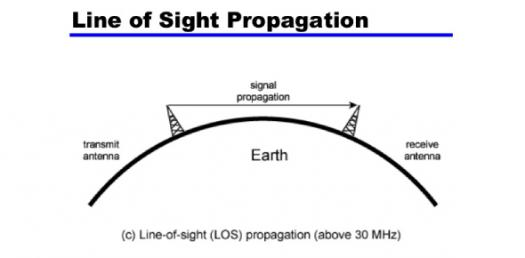# Antenna And Wave Propagation Questions! Trivia Quiz

32 Questions | Attempts: 1515
ShareSettingsAn antenna is an electrical conductor or system of conductors. Wave propagation is any of the ways in which waves travel. Having covered the process in class these past weeks you are now well capable of taking up the quiz below with ease. Give it a try and share your score.

• 1.
The unit of attenuation is
• A.

DB/m

• B.

V/m

• C.

Amp/m

• D.

Coulomb/m

• 2.
At f=30MHz, the length of the mobile whip antenna is
• A.

0.4572m

• B.

4.572m

• C.

45.72m

• D.

0.4572m

• 3.
The length of the mobile antenna is
• A.
• B.
• C.
• D.
• 4.
In a travelling wave antenna if the length of the wire increases the major lobes
• A.

Become closer to the wire axis

• B.

Become vertical to the wire axis

• C.

Move away from the wire axis

• D.

Do not appear

• 5.
A transmission line can be converted into
• A.

A dipole antenna

• B.

A dish antenna

• C.

A horn antenna

• D.

Lens

• 6.
• A.

Horizontal

• B.

Elliptical

• C.

Vertical

• D.

Nil

• 7.
An EM wave when incident on a perfect conductor is
• A.

Reflected completely

• B.

Transmitted completely

• C.

Reflected and transmitted

• D.

Refracted completely

• 8.
The tangential electric field at a perfect conductor is
• A.

1

• B.

Infinity

• C.

-infinity

• D.

0

• 9.
The critical frequency of the ionospheric layer of electron density N is
• A.
• B.
• C.
• D.
• 10.
The permitivitty of space is
• A.

1

• B.
• C.

>1

• D.

Infinity

• 11.
When a wave propagating in a free space enters the ionosphere, its velocity
• A.

Becomes 0

• B.

Increases

• C.

Decreases

• D.

Constant

• 12.
The surface current density of a good dielectric medium is
• A.

0

• B.

Infinity

• C.

Finite

• D.

-1

• 13.
The characteristics of a good dielectric depends on
• A.
• B.
• C.
• D.

F only

• 14.
The conducting properties of a good dielectric depends on
• A.
• B.
• C.
• D.

F only

• 15.
The unit of permitivitty is
• A.

• B.

Henry

• C.

• D.

Henry/m

• 16.
The unit of propagation is
• A.

Nil

• B.

1/m

• C.

1/m2

• D.

• 17.
E.H for a plane wave is
• A.

0

• B.

1

• C.

Does not exist

• D.

EH

• 18.
The unit of depth of penetration is
• A.

DB

• B.

M

• C.

Neper

• D.

• 19.
The polarization of horizontal dipole is
• A.

Vertical

• B.

Horizontal

• C.
• D.

Elliptical

• 20.
The poynting vector has unit of
• A.

Watts/m3

• B.

Watts

• C.

Watts/m2

• D.

Volt-ampere

• 21.
Ground wave propagation is affected by
• A.

Weather condition

• B.

Rain

• C.

Temparature changes

• D.

Ground constant

• 22.
The relative permitivity of the ionosphere is
• A.

More than 1

• B.

Equal to 1

• C.

Equal to infinity

• D.

Less than 1

• 23.
Troposcatter communication is used at
• A.

UHF and VHF

• B.

LF

• C.

HF

• D.

VLF

• 24.
If the direction of propagation of an electromagnetic wave is in z-direction,the polarization is in
• A.

Z-direction

• B.

Y-direction

• C.

X-direction

• D.

Circular polarization

• 25.
Directivity is
• A.

Inversely proportional to beam width

• B.

Inversely proportional to square of beam width

• C.

Directy proportional to beam width

• D.

Directly proportional to square of beam width

## Related TopicsBack to top
×

Wait!
Here's an interesting quiz for you.